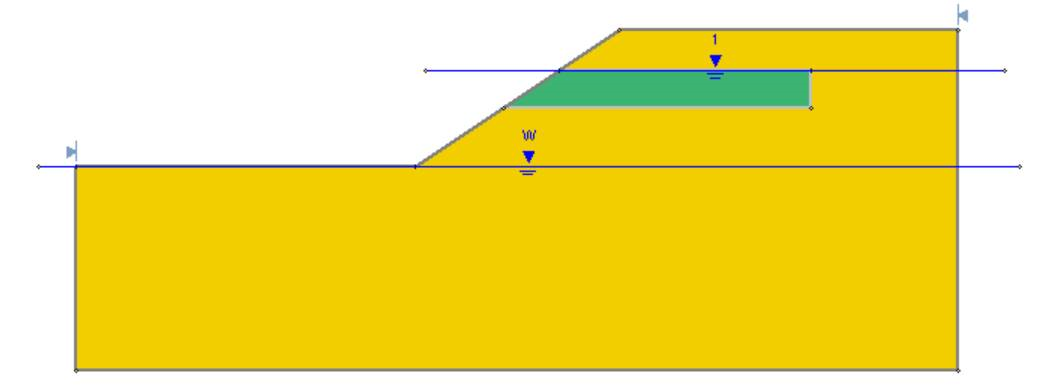The Rocscience International Conference 2021 Proceedings are now available. Read Now

# Groundwater

How can I measure flow through a dam or out a drain?

In a FEA seepage analysis, the flow rate can be determined using a series of discharge sections.

The flow rate out of a drain can be measured by putting discharge sections around the drain, but a better way to do it is to compare the discharge before and after the drain. The difference in discharge values across the body of the dam will give you the discharge through the drain.

When drawing discharge sections, make sure that they are drawn across the elements and not on a material boundary.

What does the Water Table Location sensitivity plot show?

The water table location is simply a variable normalized to the range of 0 to 1, where 0 represents that minimum water table location, and 1 represents the maximum water table location. Intermediate water tables are generated by varying the location of vertices along vertical lines, between the min and max water tables. So, if the water table location = 0.5 for example, this would represent a water table half way between the min and max water tables (i.e. if you drew a vertical line between the min and max water tables at any point, a water table location of 0.5 would have a y-coordinate half way between the min and max water tables at that x-location.

This is a simple method of generating water tables between min and max values.

There is currently no method of viewing the intermediate water tables which are generated, or obtaining their coordinates. You could do this manually, by using the water table location variable, to determine the actual coordinates.

See the Water Table Statistics help topic and tutorial for more information.

How can I model a lined pond (account for weight of ponded water, with zero pore pressure in slope)?

To account for the weight of the ponded water, while having zero pore pressure in the slope, set the Water Surface to None in the Define Materials Properties dialog.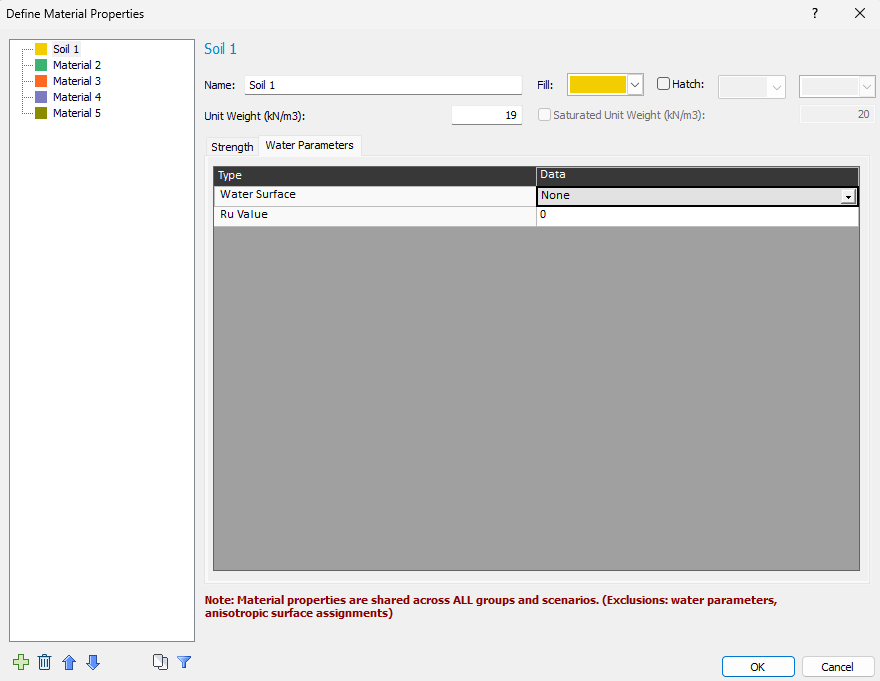Define Material Properties dialog

If the water table is above the ground surface, ponded water is automatically created and the weight of the water is added to each slice, regardless of whether it creates pore pressure in a material. To see this, simply look at the slice weights by turning on the slices.

If you want a different pore pressure distribution in the foundation material then what would be given by the water table, you need to use piezometric lines. You define a piezometric polyline in a similar way as the water table but at a different location. You can then apply that piezometric line to any material. To add a piezometric line, go to the boundaries menu in the Slide2 modeler.

Are flow quantities presented per unit length?

All flow quantities are per unit length out of plane (into the computer screen). So, if your slope was 10m long out of plane (into the computer screen), you would have to multiply the flow values by 10 to get the actual amounts.

The same applies for pump values (with withdrawal rates in units of volume per time). All results are per unit distance out of plane. This would be like having an infinite line of pumps separated by a meter out of plane. If you have some out of plane spacing, you have to account for this in the values you use for flow/pump rates. For example, if the out of plane spacing is 2m, you would divide the pump rate by the out of plane spacing (2m) to get the pump rate per meter out of plane.

When using a pore pressure grid, is there a way to exclude a material?

When using a pore pressure grid, if you do not want pore pressure in a particular material, simply turn off the grid in the material properties dialog.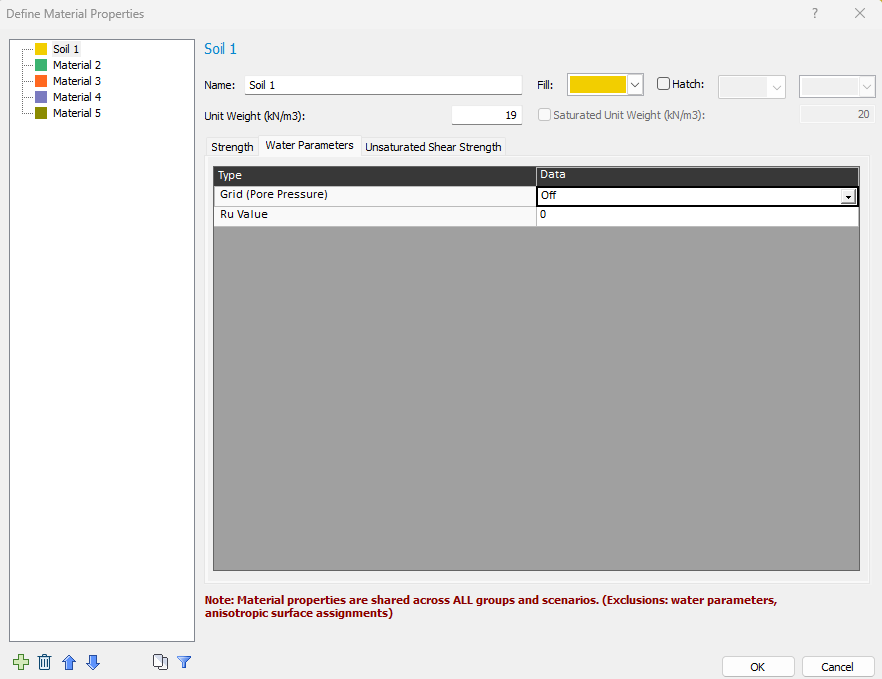Option to turn off pore pressure grid in material

If the Grid (Pore Pressure) option is On, pore pressures will be computed from the grid for any surface going through that material. Values outside the pore pressure grids will be extrapolated.

What is the difference between a piezo line and a water table?

Piezo lines are generally used when there are multiple water tables for different materials or when there is an artesian condition for one of the materials.

The method of pore pressure calculation is exactly the same for a Water Table or a Piezometric Line. However, Piezometric Lines are differentiated from the Water Table in Slide2 for the following reasons:

The Water Table can be used to define ponded water, by simply drawing the Water Table above the External Boundary. A Piezometric Line drawn above the External Boundary, will NOT define ponded water.

A Water Table allows you to define different Unit Weights for a material above and below the Water Table. This cannot be done with a Piezometric Line.

A Water Table can be used in conjunction with a Pore Pressure grid, to ensure that pore pressures above the Water Table are always zero. (See the Water Pressure Grid topic for details.) Piezometric Lines cannot be defined in conjunction with Water Pressure Grids in Slide2.

Is a total or effective stress method better for stability computations?

You can use either a total or effective stress method of doing stability computations and you should get similar results. Which you use is up to you and depends on which method you are more comfortable with and what properties you have (drained versus undrained). For rapid drawdown, we also offer both a total stress and effective stress option, we’ve seen different customers use one or the other or both.

Hydraulic Boundary Conditions

This article describes the different hydraulic boundary conditions available in Slide2, and should make it more clear when each of the boundary conditions would be best used.

The figure below illustrates many of the different boundary conditions:

No flow: HI

Free surface: CD

Note: The location of D is determined by the program as part of the solution, it is not defined by the user. It is the boundary between the saturated and unsaturated zones.

Seepage Face: DE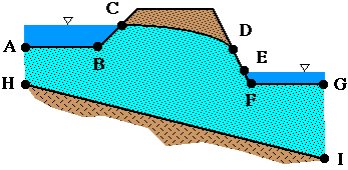Descriptions of each boundary condition can be found below:

Constant Head: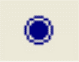• Head at a given location does not change
• Implies an inexhaustible supply of water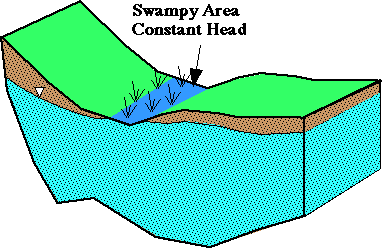Nodal Flow:• Constant rate of injection/extraction of water
• Head is calculated to produce specified flow (resulting head may be above ground surface or below base unless checked)
• This is analogous to a point load in FEM.

Seepage Face:• Means that total head (h) must never exceed elevation head (z)
• Saturated zone intersects ground surface at atmospheric pressure and water discharges as evaporation or downhill film of flow
• General location of surface is known but its length is unknown prior to numerical analysis
• Modeled using the Unknown (Q=0 or P=0) boundary condition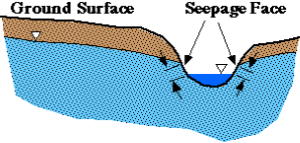Infiltration: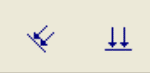• Volume of water entering or leaving an area (e.g. rainfall)
• Can only be applied to line segments and not individual nodes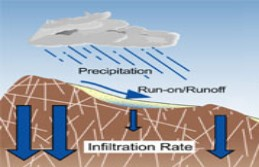No Flow:• Similar to nodal flow except flow at boundary equals zero
• May be used to represent boundaries that yield negligible amounts of water to system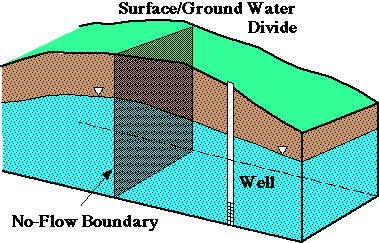Also note the following tips regarding specifying boundary conditions:

• When a boundary has no boundary conditions assigned to it, that boundary is an impermeable boundary.
• When assigning a "Seepage Face condition" or "Unknown Boundary Condition" for a node or a segment of a boundary, that section cannot have pressure greater than zero. This means that whenever the water table rises to the level of that point, water can flow out of the model from that point (seep out).
• Discharge sections are best put inside boundaries and not on them.

Does Slide2 incorporate initial water content into the groundwater formulation?

The saturation (or volumetric water content) is not included in the formulation of groundwater flow. For the case of unsaturated steady state flow, the “permeability coefficient” is dependent on the matric suction in the unsaturated zones. In transient cases the variation of volumetric water content with matric suction is taken into account just to evaluate the “storage coefficient” used in the formulation.

Can I import a PWP grid for a low k material, and then run a slope stability analysis, instead of using the b-bar method to determine pore pressures?

It’s quite common that people will use a finite-element product to compute excess pore pressure and then import this into Slide2 as a pore pressure grid. As long as the pore pressure distribution is correct, and make sure you check it using the Supplemental contours option in the Data menu of the Interpreter, the slip surfaces will be valid. This also assumes your effective strength criterion is correct.

How do I incorporate data from piezometers installed in the field?

The output from a seepage analysis is total heads (or pore pressures) throughout the model. So when you have pore pressures at particular points, as you do with piezometer readings, you want to correlate the results of your model with the measured piezometer readings. So you end up modifying your groundwater model in terms of permeability’s, permeability model (i.e. simple, van Genuchten, Brooks and Cory), and far field boundary conditions, until you get a fit between your model and the pore pressures measured at certain locations.

What some customers do incorrectly, is apply pressure boundary conditions at the points where the piezometers are located. Although this forces the pressure at these points to be the measured values, this also creates a source or a sink at these locations resulting in fluid being injected or removed at these locations.

B-bar analysis modeling tips

The following tips are good to keep in mind when carrying out excess pore water pressure analyses in Slide2:

1. Excess pore pressure models are really two stage models. If a materials weight creates excess pore pressure (checkbox in the material properties dialogue) then it’s assumed that this material is added to the system in the second stage (like an embankment in Tutorial 12) and its weight creates pore pressure in an underlying material. If the materials weight does not create excess pore pressure, it’s assumed to exist in the first stage. Second stage materials lying above it will generate excess pore pressure if their weight is defined as creating excess pore pressure.
2. If a material in Slide2 has zero initial pore pressure, it is assumed to be unsaturated and any load will not create an excess pore pressure. So if Ru is 0, no excess pore pressure will be created. To get around this, define a very small value of Ru (say 0.00001). This will trick the program into having almost zero initial pore pressure for a material yet adding excess pore pressure.
3. If you are doing a B-Bar analysis then you should be using effective parameters. See Tutorial 13, all the materials use effective values with a water table defined. Whether it’s rapid drawdown or a loading condition that creates the excess pore pressure, you use effective stress parameters. See Tutorial 12 for an example of an embankment load creating excess pore pressure.
4. Excess pore pressure is only created in the saturated zone. The saturated zone is any area in which the initial pore pressure is greater than zero.

General groundwater analysis modeling tips

The following are general guidelines and tips that should be kept in mind for Slide2 groundwater analyses.

1. In general, large contrast in permeability for two adjacent material cause numerical instability. The use of appropriate hydraulic permeability function solves some of these problems. Refining the mesh may also help alleviate numerical issues.
2. The “Simple” permeability function is only meant to give you reasonable results for the phreatic surface location for steady-state seepage analysis. For more realistic groundwater results you need to use actual permeability function or more sophisticated hydraulic conductivity functions such as the Van Genutchen or Gardiner models.
3. The transient analysis requires an initial state of the pore water distribution in the model. The starting point is crucial for the stability and convergence of the solution.
4. For the case of initial dry soil, the precision of the water content curve plays the major role that affects the solution. Therefore, a rough and preliminary model like the “Simple” function is not suitable for such analysis.
5. The groundwater compute engine in Slide2 solves steady-state and/or transient seepage analysis as a standalone module without the need to do any slope stability analysis after.
6. The FEA seepage analysis in Slide2 is very powerful and useful tool for analyzing seepage flow and discharge quantity. Lack of field data for any numerical analysis reduces its usefulness. But, these tools can be used to explore what if scenarios and check several cases of interest. In seepage analysis, you might have some idea on water level at specific location at your site from standpipes or wells which will be a start-up location to specify the required boundary conditions for the model.

How can I model a well that pumps water out of a system?

This can be modeled using a negative nodal flow boundary condition or negative infiltration.

How do I model aquifer storage in Slide2?

The storage quantity can be calculated based on other input terms from Slide2 (mw and the water content curve). The slope of the water content curve that Slide2 requires in the transient analysis is mw (coefficient of volume change).

Aquifer storage is shown in Verification Example #21.Comparison of Slide2 results and Analytical Solution for Transient Seepage through Fully Confined Aquifer with no initial pore-water distribution

How can I model an excavated boundary condition in Slide2?

Modeling an excavated boundary condition in Slide2 is complex. The example shown below was done using transient boundary conditions that consider changes of total head with time and applied them on different elevation levels. The changes of total head followed the 1m interval of the excavation steps in the example.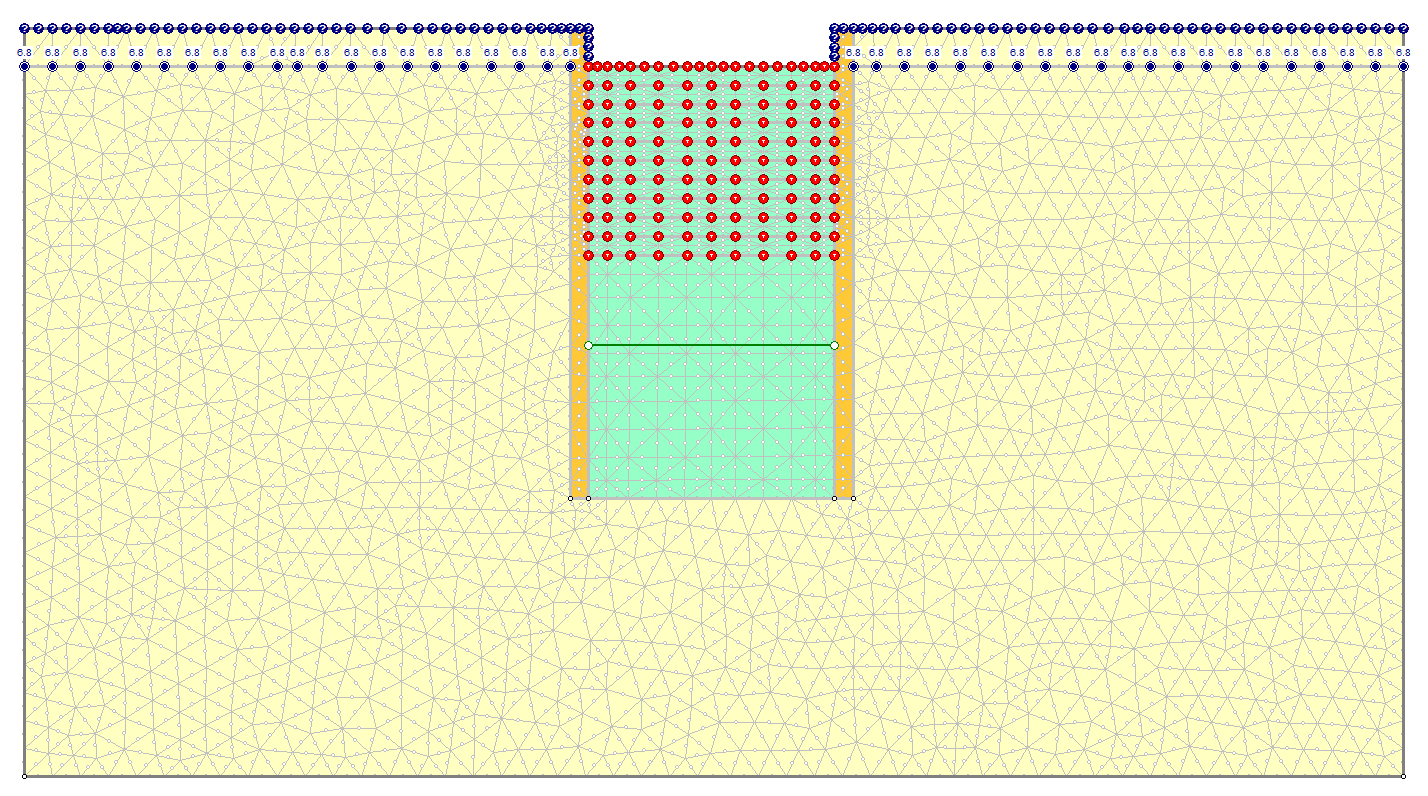Example of excavated boundary condition in Slide2

Why is there flow in the unsaturated zone of the model?

Users often ask why they have flow above the phreatic surface (i.e. Flow vectors in the unsaturated zone in Interpret).

The reason for this is that Slide2 performs a saturated-unsaturated steady-state seepage analysis that assumes flow above the phreatic surface. Below the water table, the degree of saturation is 100% while above the water table, the degree of saturation is less than 100%, but not 0%. Since there is water above the water table, there is still some flow. Usually the quantity of flow is small due to a reduced permeability. The permeability above the water table is defined using the permeability function defined in the groundwater properties dialog.

Fredlund, D.G. and Rahardjo, H. (1993). "Soil Mechanics for Unsaturated Soils." John Wiley, New York.

Is there a way to query the values of the pore water pressure on the contours?

Selecting the View>Data Tips>Query Datatips option in the Interpreter will allow you to move your cursor around the contours to see values.

How can I delete or move a water table boundary which exactly overlaps another boundary (e.g. a material boundary)? When I try to edit the water table, both boundaries get selected.

Use the Selection Filter option. This allows you to choose which boundaries can be selected for editing purposes. For example:

1. After you have selected an editing option (e.g. Delete Boundary or Move Vertices), right-click the mouse and choose Selection Filter from the popup menu.
2. Use the checkboxes in the dialog to choose which boundary types can be selected (e.g. Water Table). Select OK.
3. Now you can select and edit only the desired boundary type(s), without affecting other boundaries which may be overlapping or adjacent.

TIP: you can also RIGHT-CLICK directly on a Water Table and select Move Boundary or Delete Boundary from the popup menu, to move or delete the entire Water Table without affecting other boundaries. (If you want to edit individual vertices which overlap another boundary, then you must use the Selection Filter option.)

How can I model an impermeable (zero permeability) material for a finite element groundwater seepage analysis? For example, an impermeable sheet pile wall.

A material with zero permeability (Ks = 0) cannot be explicitly modeled in a Slide2 seepage analysis. If you specify a zero permeability material, this will cause numerical problems with the finite element analysis, and must be avoided.

However, an impermeable material can be simulated simply by specifying a very low value of permeability Ks. For example, if you specify a permeability which is several orders of magnitude smaller than the surrounding materials (e.g. 1e-20 m/s), then this will effectively simulate a zero permeability material. The flow through the low permeability material will be negligible, compared to the flow through the surrounding, higher permeability material.

In a future version of Slide2, an explicit zero permeability material or boundary condition may be added for the finite element seepage analysis. However, in the mean time, the above suggested method works very well, and is also very simple to model.

For a tutorial which illustrates this method (for sheet pile walls in a cofferdam), see Tutorial 14 - Cofferdam Seepage.

## Theory

How does Slide2 incorporate compressibility in transient seepage analyses?

The slope of the water content curve that Slide2 requires in the transient analysis is mw (coefficient of volume change).

What does Slide2 do with pressure head?

Slide2 converts pressure head to pore pressure by multiplying by the unit weight of water. Then uses pore pressure to determine effective normal stress on the base of a slice. In the attached hand calculation, u is the pore pressure. It’s standard Terzaghi effective stress theory. Pore pressure is not used in the determination of interslice forces in Slide2. Slide2 uses total forces for everything, only in the determination of shear strength on the base does pore pressure get used.

How does pore pressure influence the results in a slope stability analysis?

What pore pressure does is reduce the effective stress. The effective stress being the amount force being transmitted through the soil skeleton per unit meter of area. For a constant total stress, as the pore pressure increases, the effective stress decreases. Since strength in a frictional material is a function of normal effective stress, an increase in pore pressure generally reduces strength.

What is the difference between the water table and piezometric lines?

Piezolines do not create ponded water. When you add a water table above the ground surface, ponded water is automatically added (as shown in the figure below), including the weight of the water.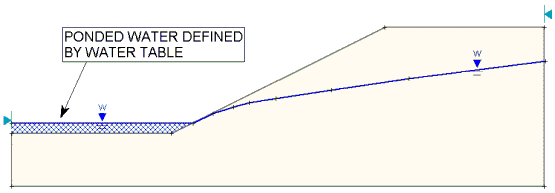Ponded water automatically created by Water Table

Details of this feature can be found in the Add Water Table section of the Online Help.

Is the factor of safety for slope stability affected by negative pore pressures?

Factor of safety is only affected by negative pore pressures from a groundwater analysis if the Phib value is nonzero. For more details see the Unsaturated Shear Strength help topic.

How can I incorporate a perched groundwater material above the water table?

To define a perched groundwater material, you need to define your water table as usual, and assign it to all relevant materials, but NOT the perched layer. You then need to define a piezometric surface above the perched layer that is assigned ONLY to the perched layer.

In the model pictured below, the perched layer is shown in green. The water table, labeled “W” is assigned to the yellow material only while the piezometric surface, labeled “1,” is assigned to the green material only.